# Collecting Like Terms

Collecting Like Terms• Like terms are the parts of an expression that have the same algebraic variable (same letter).
• Collecting like terms means to count up how many of each different letter there are in an algebraic expression.
• We combine like terms to make an expression shorter and simpler.
• To collect like terms, add up the numbers in front of each letter separately.
• We combine the r terms: 4r + 2r = 6r.
• We don’t write the number 1 in front of letters in algebra so ‘t’ is the same as ‘1t’.
• So 3t + t = 4t.
• The expression 4r + 3t + 2r + t simplifies to 6r + 4t.

To collect like terms, add the numbers in front of the letters that are the same.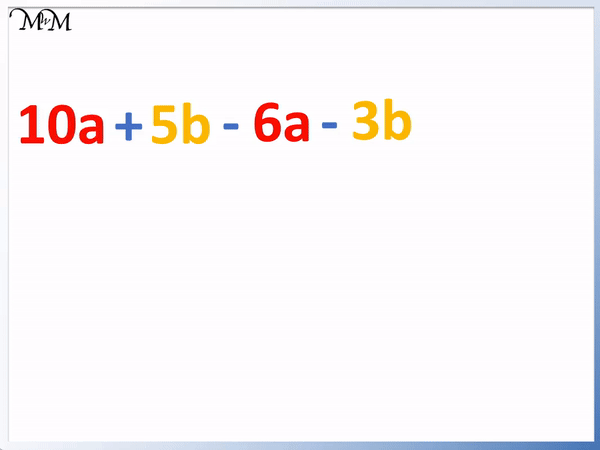• We collect the ‘a’ terms and the ‘b’ terms separately.
• The subtraction sign in front of 6a belongs in front of the 6a.
• So we have 10a – 6a, which equals 4a.
• The subtraction sign in front of the 3b belongs in front of the 3b.
• So we have 5b – 3b, which equals 2b.
• 10a + 5b – 6a – 3b simplifies to 4a + 2b.
• It can help to use different colours to show different like terms and also to rewrite the expression so that the like terms are next to each other.Supporting Lessons

#### Combining Like Terms with Negative Coefficients# Collecting Like Terms

## What are Like Terms?

Terms are the different parts of an algebraic expression that are separated by either a plus or a minus sign. Like terms are simply any of these terms that use exactly the the same letters.

For example, in the expression z + a + z + b + a + b, the two a terms are like terms, the two z terms are like terms and the two b terms are like terms.

We can only add terms with the same letter. We cannot add a to b, a to z or b to z.The letters in algebra are used to represent unknown values and they are called variables.

The value of an apple can be written as using the variable ‘a’ and the value of a banana can be written using the variable ‘b’. The apple and the banana are two different items and therefore the variables ‘a’ and ‘b’ are not like terms.Like terms must have exactly the same variables. We can add up how many apples we have and how many bananas we have by counting them separately.

For example, here is a list of apples and bananas.Combining like terms is important because it allows us to write algebraic expressions more efficiently. Instead of saying that we have an apple, an apple, a banana, a banana, a banana, an apple and an apple, it easier to say that we have four apples and 3 bananas.We can replace each item with its algebraic variable.It is easier to write a + a + a + a + b + b + b as 4a + 3b. This is collecting like terms.Counting up the list of variables is called combining like terms.4a means 4 × a and 3b means 3 × b but we don’t write the multiplication signs in algebra.

Each of the 4 letter a’s in the expression a + a + a + a are all like terms.

Each of the 3 letter b’s in the expression b + b + b are all like terms as well.

Collecting like terms basically means that we cannot add a terms to b terms. Just like we count apples and bananas separately.

Here is a similar example where the variable z is used to represent the cost of a zebra toy and the variable g is used to represent the cost of a giraffe toy.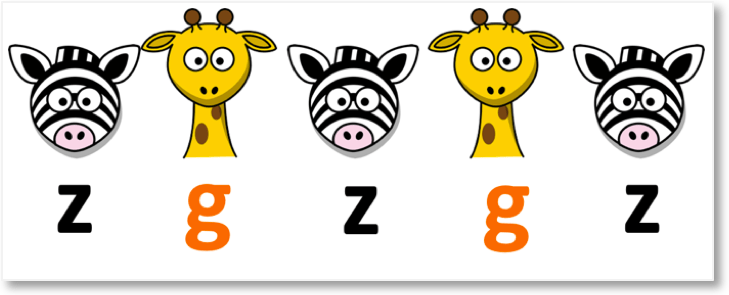It is easier to count the number of zebras and giraffes by first looking at the zebras all together and then separately looking at all of the giraffes together.The 3 z’s are all like terms and they are different to the 2 g’s which are also like terms.

z and g are different letters and so they are not like terms.Combining like terms, z + z + z + g + g = 3z + 2g.

It is much quicker to say that the total cost of the animal toys is 3z + 2g, rather than listing out all of the animals.

## What does Combining Like Terms Mean?

Combining like terms means to simplify an algebraic expression by counting the variables that are the same. Simply look at each letter in turn and add the numbers in front of them to see how many there are in total.

For example, in the expression 3a + 4c + 2a, we can combine like terms to get 5a + 4c.

It does not make sense to have two separate terms with a in them. Instead of writing 3a and 2a separately, it is easier to add 3 + 2 to get 5 and write 5a.Remember that we can only add a terms to other a terms.

We cannot add the 4c term to the 5a term, so 5a + 4c is our answer. We cannot simplify 5a + 4c any further.

The number in front of each algebraic term is called the coefficient. There can be several coefficients in a single algebraic expression and we can add them if the variable they are in front of is the same.

In this example, each cake costs ‘c’.Here we have several of these cakes in boxes. The first box has 3 cakes and so it costs 3c. The second box has 2 cakes so it costs 2c and the third box has another 3 cakes and so, it costs 3c.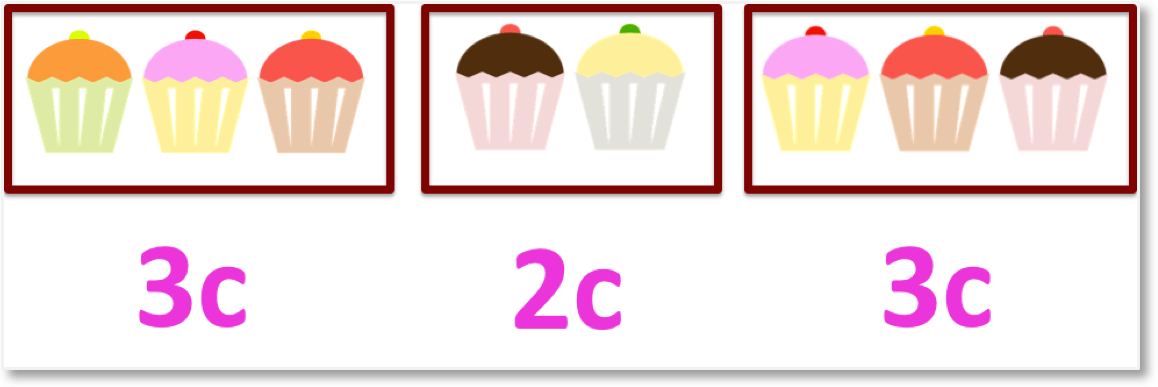It total there are 8 cakes, so we can say that the total cost is just 8c.

We can collect the like terms to write 3c + 2c + 3c as 8c.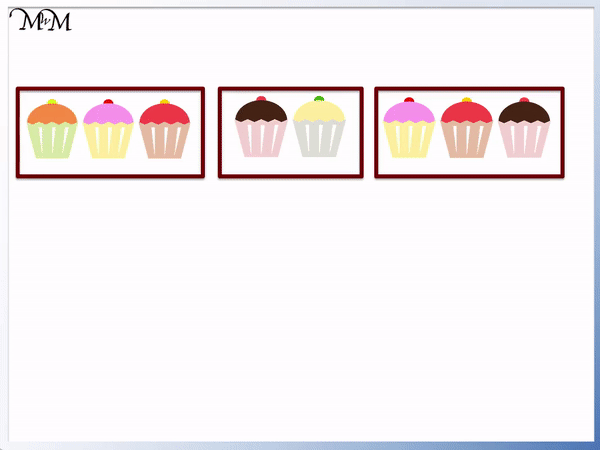We can see that because each letter is the same, we can add the numbers in front of them.

3 + 2 + 3 = 8 and so, 3c + 2c + 3c = 8c.

We need to keep the variable c in our answer.## How to Combine Like Terms

To combine like terms add the coefficients (numbers) in front of the variables (letters) that are the same. If there is no coefficient written in front of a variable, then the coefficient is actually worth 1.

We tend to write the answer with the variables in alphabetical order.

For example, here is the expression 6f + 2w + 2f + a.We can see that the first term alphabetically is ‘a’. There are no other ‘a’ terms to add it to so we just have this one. Remember that ‘a’ is the same as ‘1a’. We do not write 1 in front of a variable in algebra.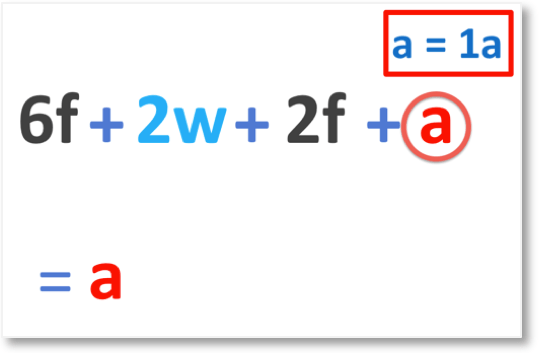Next in alphabetical order are the ‘f’ terms. We have 6f and 2f. The coefficients are the numbers in front. We have 6 and 2.

6 + 2 = 8 and so, 6f + 2f = 8f.Finally, we have the term ‘2w’ but again there are no other like terms to add it to because there are no other w’s in this expression. We can only add numbers in front of the same letters.6f + 2w + 2f + a can be written as a + 8f + 2w when we combine like terms and write the terms in alphabetical order.We simply add the numbers in front of each letter if the letters are the same.

Here are some more examples of collecting like terms with coefficients.

When teaching collecting like terms, it can be useful to use different colours to highlight different like terms.

In this example we have 4r + 3t + 2r + t.

We first look at the r terms. 4 + 2 = 6 and so, 4r + 2r = 6r.

We now look at the t terms. 3 + 1 = 4 and so, 3t + t = 4t. Remember that t is the same as 1t.Combining like terms, 4r + 3t + 2r + t = 6r + 4t.

Notice that the two terms in the answer are separated by a plus sign.

A common mistake when learning how to collect like terms is to simply list the terms. It is important that each separate like term in the answer is separated by a plus or minus sign.

Here is another example of combining like terms with coefficients.

We have b + 3z + 2b + z.Looking at the b terms, 1 + 2 = 3 and so, b + 2b = 3b.

Looking at the z terms, 3 + 1 = 4 and so, 3z + z = 4z.

Remember to separate the variables in the answer with a plus sign. We have 3b plus 4z so we write 3b + 4z.

## Combining Like Terms with Subtraction

If there is a subtraction sign in front of a term, then this number is subtracted from any other like terms. The variable still remains the same.

For example here we have 10a + 5b – 6a – 3b.

We can write the a terms together. We have 10a – 6a. Notice that we moved the minus sign with the 6a.

We write the b terms together. We have 5b – 3b. Again we move the minus sign in front of the 3b too when we move the 3b.10a – 6a = 4a and 5b – 3b = 2b.

We have two terms, 4a and 2b.

We combined like terms by subtracting the coefficients. 10a + 5b – 6a – 3b = 4a + 2b.

Notice that because 5b – 3b = 2b, we still have 2 b terms left after the subtraction, so there is a plus sign between 4a and 2b.

We can simply subtract the coefficients of each term.

Here are 8 cakes, which we can write as 8c.We will remove the box of 3 cakes to leave 5 cakes remaining.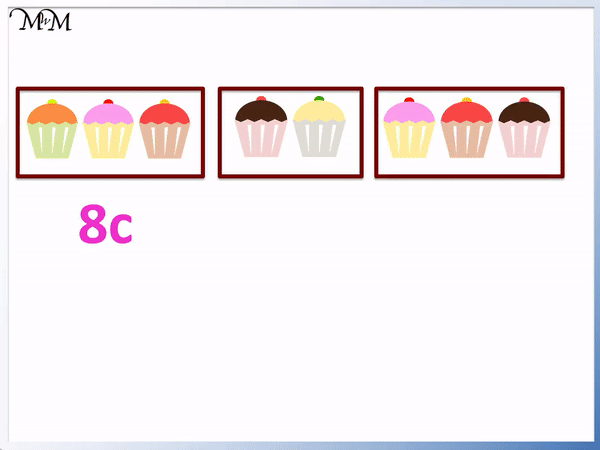We can see that 8 cakes take away 3 cakes leaves 5 cakes.

Therefore we can write 8c – 5c = 3c.We can see that 8 – 5 = 3 and so, 8c – 5c = 3c. We simply subtract the coefficients. If there is a minus sign in front of a term, then we subtract the number.

He is a simple example of combining like terms using subtraction.

We have 4a – 2a.

We can see that because both terms contain an ‘a’ and only an ‘a’, we have like terms.

We can subtract the coefficient of 2 from the coefficient of 4.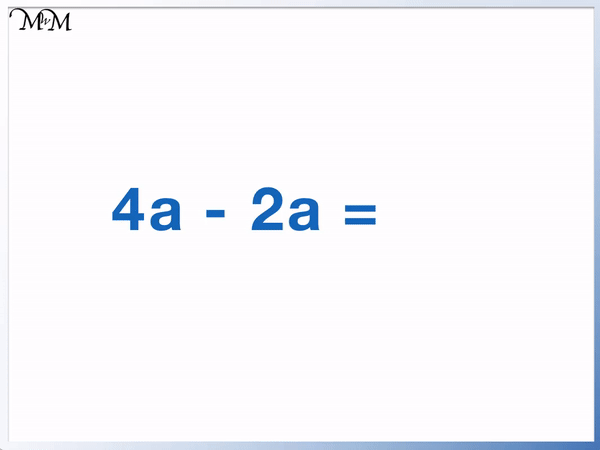4 – 2 = 2 and so, 4a – 2a = 2a.

We can think of this as a negative coefficient. We have 4a and -2a. The coefficient of the second a term is -2.

When teaching collecting like terms with negative coefficients, it is important to show that any minus signs in the expression belong to the following term.

For example, here we have 4a – 2a + 5b – 3b.

There are two minus signs in this expression.

The first minus sign belongs to the 2a and the second minus sign belongs to the 3b.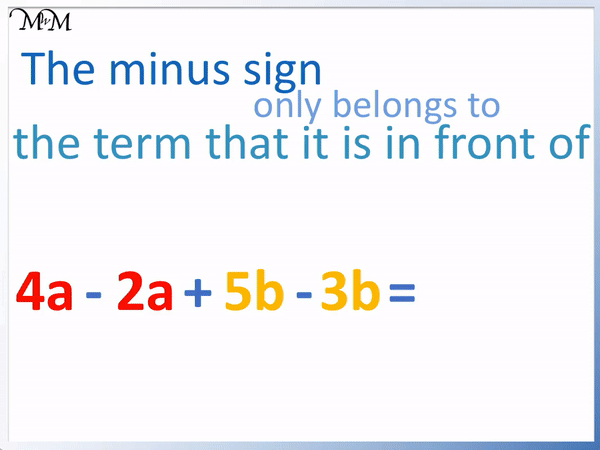4 – 2 = 2 and so, 4a – 2a = 2a.

5 – 3 = 2 and so, 5b – 3b = 2b.

We have 2a + 2b.

Notice that we still have a plus sign separating the terms in our answer because we have + 2a and + 2b. We did not lose any more than we started with so we do not have any negatives in our answer.

Here is an example of 5p + 2r – 3p – r.We can simplify this expression by writing the like terms together.

We will write the p terms together. Notice that we move the minus sign with the – 3p term.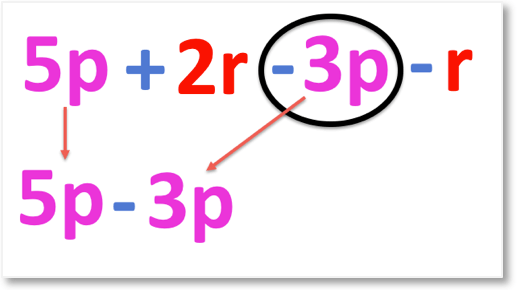We then gather the r terms, making sure that we keep the minus sign in front of the – r term.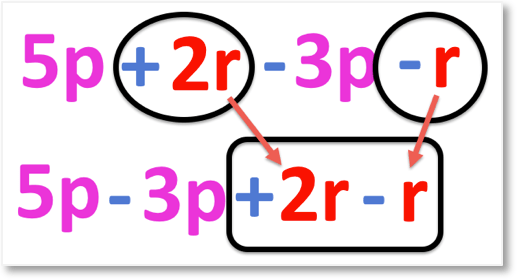Now collecting the p terms, 5 – 3 = 2 and so, 5p – 3p = 2p.Now collecting the r terms, 2 – 1 = 1 and so, 2r – r = r.We can see that 5p + 2r – 3p – r = 2p + r.Here is another example of 4d + 7h – 4d.We first look at the d terms. 4d – 4d = 0d.

We do not write 0d. Because there are no d terms remaining, then we do not write anything.

It is a common mistake for students to write a 0 as a coefficient after a subtraction has occurred. We should never write any terms that have a coefficient of 0 because it adds unnecessary information.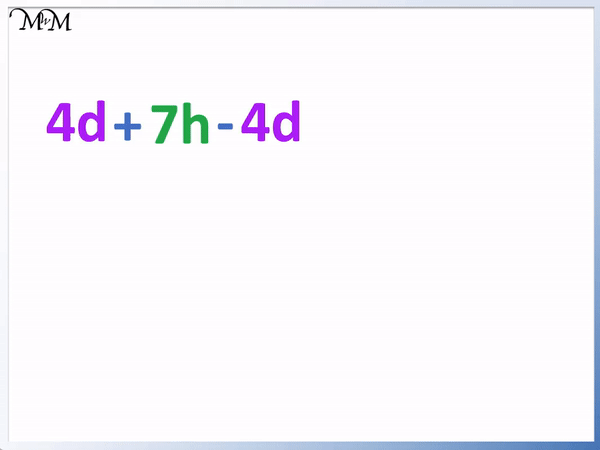4d + 7h – 4d is simply written as 7h. We only have 7h remaining after subtracting the 4d term.

Here is another example of simplifying an algebraic expression by cancelling terms. We have 3e + 2s – 3e – s.

Looking at the e terms, 3e – 3e = 0e. There are no e terms left so we do not write them.We only have the s terms left.

2s – s = s.

So after combining like terms, 3e + 2s – 3e – s = s.

We can see that it is much easier to say that we have s instead of listing the much longer and more complex original expression.Now try our lesson What is a Positive Plus a Negative? where we learn how to add a negative number.error: Content is protected !!